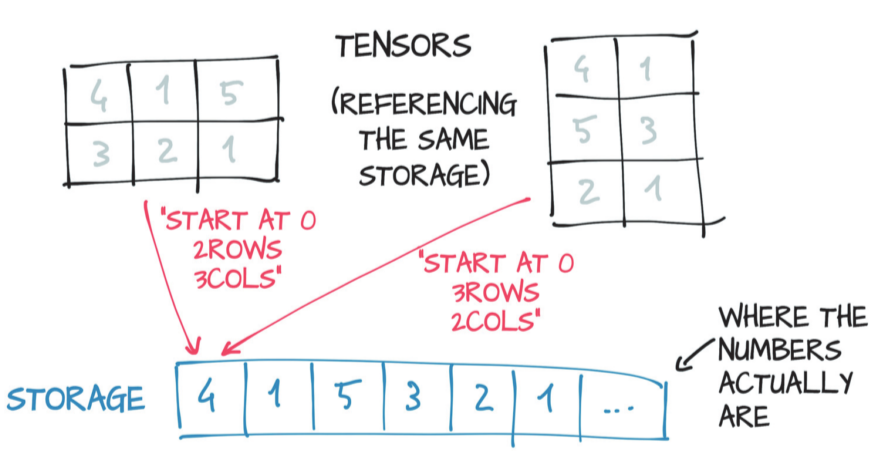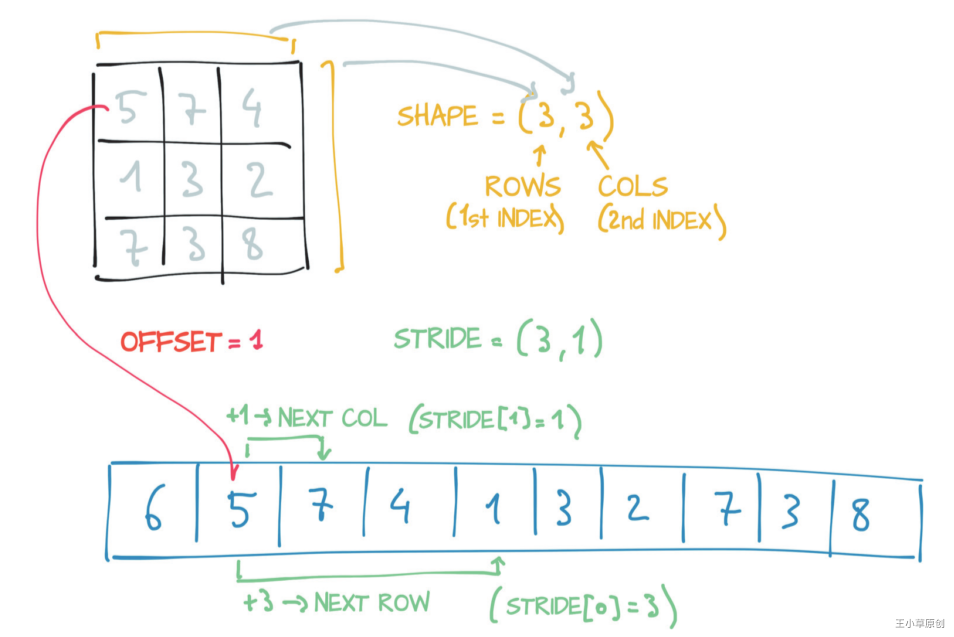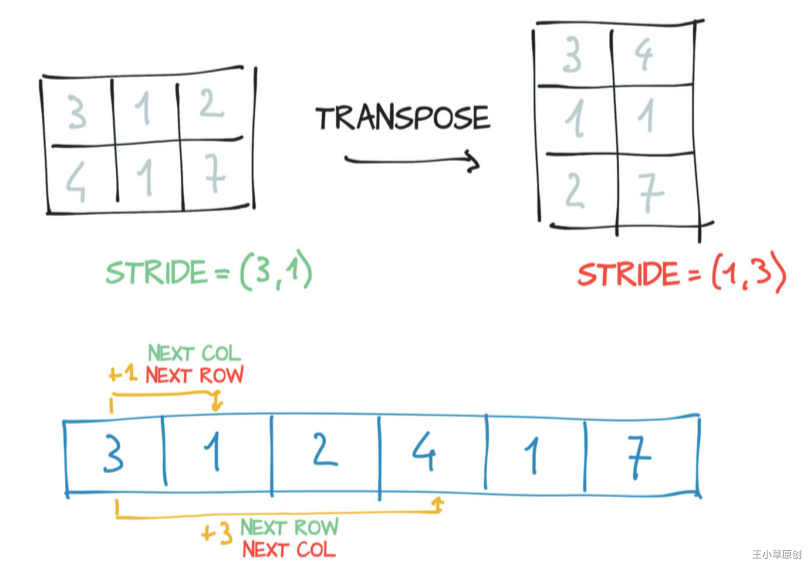# 2.4 Tensor的存储

• 第二章：认识Tensor的类型、创建、存储、api等，打好Tensor的基础，是进行PyTorch深度学习实践的重中之重的基础。
• 第三章：学习PyTorch如何读入各种外部数据
• 第四章：利用PyTorch从头到尾创建、训练、评估一个模型，理解与熟悉PyTorch实现模型的每个步骤，用到的模块与方法。
• 第五章：学习如何利用PyTorch提供的3种方法去创建各种模型结构。
• 第六章：利用PyTorch实现简单与经典的模型全过程:简单二分类、手写字体识别、词向量的实现、自编码器实现。
• 第七章利用PyTorch实现复杂模型：翻译机（nlp领域）、生成对抗网络（GAN)、强化学习(RL)、风格迁移（cv领域）。
• 第八章：PyTorch的其他高级用法：模型在不同框架之间的迁移、可视化、多个GPU并行计算。

### 2.4.1 Storage

tensor中的值是存储在连续的内存块中的，由torch.Storage实例管理着。一个storage即是一个一维的向量。import torch
# 3*2的tensor
points = torch.tensor([[1.0, 4.0],[2.0, 1.0],[3.0, 5.0]])
points.storage()

 1.0
4.0
2.0
1.0
3.0
5.0
[torch.FloatStorage of size 6]


points_storage = points.storage()
points_storage

1.0


tensor的值存储在storage中，若改变了storage的值，势必会改变tensor的值。

points_storage = points.storage()
points_storage = 2.0
print(points)

tensor([[2., 4.],
[2., 1.],
[3., 5.]])


### 2.4.2 size

size（在numpy中叫shape) 是一个元组，显示tensor中每个维度的元素数量。这个大家自然是不陌生，如：

points = torch.tensor([[1.0, 4.0],[2.0, 1.0],[3.0, 5.0]])
print(points.size())  # 打印size

torch.Size([3, 2])


### 2.4.3 storage offset

storage offset是指tensor的第一个元素在storage中的位置，再来看之前的例子

# 3*2的tensor
points = torch.tensor([[1.0, 4.0],[2.0, 1.0],[3.0, 5.0]])
points.storage()

 1.0
4.0
2.0
1.0
3.0
5.0
[torch.FloatStorage of size 6]


points_second = points
print(points_second.storage_offset())

2


points_second应为：tensor([2., 1.]), 它的第一个元素在storage中位置为索引为2的地方。

### 2.4.4 stridepoints = torch.Tensor([[5,7,4],[1,3,2],[7,3,8]])
points.stride()

(3, 1)


storage_offset + stride * i + stride * j

### 2.4.5 获取子tensor

# 创建一个3*2的tensor
points = torch.tensor([[1.0, 4.0],[2.0, 1.0],[3.0, 5.0]])
print(points.size())  # (3,2)
print(points.stride())  # (1,2)
print(points.storage_offset())  # 0

# 创建Points的子tensor
points_second = points
print(points_second.size())  # 2
print(points_second.stride()) # (1,)
print(points_second.storage_offset())  # 2

torch.Size([3, 2])
(2, 1)
0
torch.Size()
(1,)
2


# 更改子tensor的值
points_second = 10.0
print(points) # 原tensor的值也会随之改变

tensor([[ 1.,  4.],
[10.,  1.],
[ 3.,  5.]])


second_points = points.clone()


### 2.4.6 转置tensor

# 创建一个3*2的tensor
points = torch.tensor([[1.0, 4.0],[2.0, 1.0],[3.0, 5.0]])
print(points.size())  # (3,2)
print(points.stride())  # (1,2)
print(points.storage_offset())  # 0

# 创建Points的子tensor
points_t = points.t()

id(points.storage()) == id(points_t.storage())  # True, 转置后storage同一个

print(points_t.size())  # (2,3)
print(points_t.stride()) # (2,1)
print(points_t.storage_offset())  # 0

torch.Size([3, 2])
(2, 1)
0
torch.Size([2, 3])
(1, 2)
0some_tensor = torch.ones(3,4,5) # 创建3*4*5的tensor
print(some_tensor.size()) # (3,4,5)
print(some_tensor.stride()) # (20, 5, 1)

some_tensor_t = some_tensor.transpose(0,2) # 将第0维于第2维互换
print(some_tensor_t.size()) # (5,4,3)
print(some_tensor_t.stride()) # (1, 5, 20)

torch.Size([3, 4, 5])
(20, 5, 1)
torch.Size([5, 4, 3])
(1, 5, 20)


### 2.4.7 连续型Tensor

# 创建一个3*2的tensor
points = torch.tensor([[1.0, 4.0],[2.0, 1.0],[3.0, 5.0]])
print(points.size())  # (3,2)
print(points.stride())  # (1,2)
print(points.storage_offset())  # 0

# 创建Points的子tensor
points_t_c = points.t().contiguous()

torch.Size([3, 2])
(2, 1)
0


### 2.4.8 总结

01-037893
05-21416412-292万+
04-188528
11-22932
02-25728
04-241310
04-301万+
11-281581
01-0864